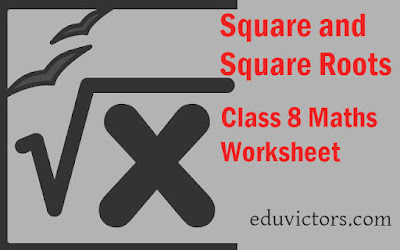# Class 8 - Maths - Square and Square Roots (Worksheet)

Q1. What will be the unit digit of the square of the following numbers?
(a) 67
(b) 23

(a) 9 (∵ 7 × 7 = 49)
(b) 9 (∵ 3 × 3 = 9)

Q2. Find the sum of 1+3+5+7+9 without adding.

Answer: 1+3+5+7+9 = 5² = 25

Q3. Fill in the blanks:
(a) The digit at unit place of square of 239 is ________.
(b) The sum of first five odd number is __________.
(c) If $6^𝑥 = 1296$, then x = .
(d) The perfect square number between 100 and 150 are ________ and ________.
(e) The number of zeroes in square of 600 is ________.

(a) 1
(b) 25
(c) x = 4
$6^𝑥 = 6^4$ ⇒ x = 4
(d) 121 and 144
(e) 4

Q4.  Find the square root using long division method
(a) 33.64 (b) 4225

Answer: (a) $\sqrt{33.64} = 5.8$

(b) $\sqrt{4225} = 65$

Q5. Find the smallest square number which is divisible by 4, 12 and 16.

Answer: For any number to be exactly divisible by 4,12, and 16, that number has to be exactly divisible by the L.C.M. of these three numbers.

LCM of 4, 12 and 16 is:

LCM = 2 × 2 × 2 × 2 × 3

Since LCM is not a perfect square. Multiply by 3 to make it perfect square.

Required number = 2 × 2 × 2 × 2 × 3 × 3 = 144 = 12²

Thus 144 is the smallest square number which is divisible by 4, 12, and 16

Q6. Find the smallest square number by which 192 must be multiplied to make it a perfect square. Also write the square root of the new number so formed.

Answer: Prime factors of 192 = 2 × 2 × 2 × 2 × 2 × 2 × 2 × 2 × 3

Since 2 and 3 is not in pair, to make perfect square multiple by 3.

= 2 × 2 × 2 × 2 × 2 × 2 × 2 × 2 × 3 × 3
= 576

$\sqrt{576} = 2 × 2 × 2 × 2 × 3 = 24$

Thus 192 must be multiplied by 3 to make it perfect square 576. $\sqrt{576} = 24$

Q7. Find the side of a square whose area is 196 sq. cm.

Answer: Let a = side of a square.

Area = a² = 196

⇒ a = $\sqrt{196}$

⇒ a = $\sqrt{2 \times 2 \times 7 \times 7}$

⇒ a = 14 units.

Q8. Find the square root of 16 and 121 using repeated subtraction method.

(a) 16 - 1 = 15
15 - 3 = 12
12 - 5 = 7
7 - 7 = 0
∴ Number of steps = 4
∴ $\sqrt{16} = 4$

(b) 121 − 1 = 120
120 − 3 = 117
117 − 5 = 112
112 − 7 = 105
105 − 9 =  96
96 − 11 = 85
85 − 13 = 72
72 − 15 = 57
57 − 17 = 40
40 − 19 = 21
21 − 21 = 0
∴ Number of steps = 11
∴ $\sqrt{121} = 11$